## SBT Prize at the end of Chapter 5 (C5 Math 7 – Horizon) – Math Book

SBT Prize at the end of Chapter 5 (C5 Math 7 – Horizon)
————-

### Solve problem 1 page 114 SBT Math 7 Creative horizon episode 1 – CTST

Learning about the ability of students to cook their own rice in class 7C is given by the following statistics:

 The ability to cook rice yourself Not achieved Obtain Good Excellent The number you rate yourself 20 ten 6 4

a) Please classify the data in the above statistical table based on qualitative and quantitative criteria.

b) Calculate the size of the class.

Detailed instructions for solving Lesson 1

Solution method

We rely on the definition of qualitative data as data represented by words, letters, symbols, names, etc.

From the above definition, we show the data in the table.

Detailed explanation

a) Qualitative data is data represented by words, letters, symbols, names, etc.

Quantitative data is data represented by real numbers.

So the qualitative data in the statistics table is the ability of the students to cook their own rice: fail, pass, good, excellent.

The quantitative data in the statistics table is a number you rate yourself: 20, 10, 6, 4.

b) The total number of self-assessed students is:

20 + 10 + 6 + 4 = 40 (you).

So the size of class 7C is 40 students.

–>

— *****

### Solve problem 2 pages 114 SBT Math 7 Creative horizon episode 1 – CTST

Find out about the interest in volleyball of 7C students as given by the following statistics:

 Attitude Dislike Not interested Prefer Like so much Number of female friends twelfth 8 2 first

Are the above data representative of the football preferences of all 7C students?

Detailed instructions for solving Lesson 2

Solution method

We rely on the data on the table to see if they have the same qualitative criteria

Detailed explanation

Since the statistics of girls in class 7C like volleyball, it is not representative of all students in class 7C’s fondness for football because they do not have the same qualitative criteria.

–>

— *****

### Solve problem 3 pages 114 SBT Math 7 Creative horizon episode 1 – CTST

Draw a line graph to represent the data of the following statistical table:

 Number of students who achieved good marks in Literature in the first 4 months of the first semester of grade 7 Month Number of students 9 15 ten 24 11 20 twelfth 36

Detailed instructions for solving Lesson 3

Solution method

To draw a line graph representing the statistics of the number of students who achieved good marks in Literature in the first 4 months of the first semester of grade 7, we perform the following steps:

Step 1: Draw two vertical and horizontal axes at right angles to each other.

– Horizontal axis: Record the timelines (months: 9, 10, 11, 12).

– Vertical axis: Select the appropriate division for the data and write the numbers in the divisions.

Here we can choose a division interval of 5.

Step 2:

– At the September landmark on the horizontal axis, mark a point about 15 distances from the vertical datum at that time, corresponding to the division on the vertical axis.

The same goes for the rest of the time points.

– Draw straight lines connecting each pair of points corresponding to a pair of consecutive time points, we get a curved line representing the change of data over time.

Step 3: Complete the chart.

– Name the chart: Number of students who got good marks in Literature in the first 4 months of the first semester of grade 7.

– Note the data values ​​at the beginning of the line segment.

– Record units on two axes

Detailed explanation

We have the following chart: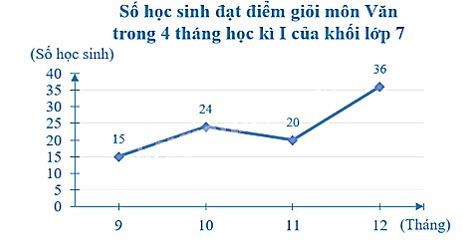–>

— *****

### Solution 4 page 115 SBT Math 7 Creative horizon episode 1 – CTST

Read the data represented in the following line graph and write it in the corresponding statistics table.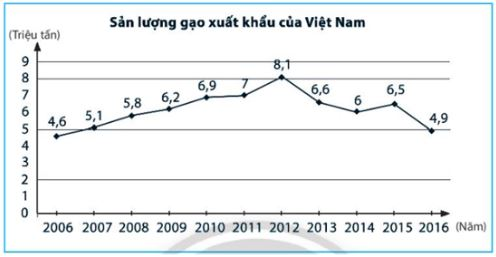Detailed instructions for solving Lesson 4

Solution method

We rely on qualitative criteria in the statistics table to read those data.

Detailed explanation

Looking at the line graph above, we see:

– The chart shows information about Vietnam’s export rice output (million tons).

The unit of time is the year.

– Rice export output of Vietnam over the years:

+ The rice export output of Vietnam in 2006 was 4.6 (million tons).

+ Similarly, we can read Vietnam’s rice export output in the remaining years.

We have the following statistics:

 Vietnam’s export rice output (million tons) Year 2006 2007 2008 2009 2010 2011 2012 two thousand and thirteen 2014 2015 2016 Quantity 4.6 5.1 5.8 6.2 6.9 7 8.1 6.6 6 6.5 4.9

–>

— *****

### Solution 5 page 115 SBT Math 7 Creative horizon episode 1 – CTST

Draw a graph to represent the data in the following statistical table:

 Nest The number of good points, good at Math of the team Percentage of good and good points of each group compared to the whole class first 28 thirty first% 2 34 38% 3 28 thirty first% Total 90 100%

Detailed instructions for solving Lesson 5

Solution method

We should determine the type of chart that we draw (should be a pie chart) then we draw with the available data in the statistics table.

Detailed explanation

To draw a pie chart representing the data as follows:

– Name the chart: Percentage of good and good points in Math of each group compared to the whole class.

– Note the object names and percentages on the chart.

Group 1: 31%; Group 2: 38%; Group 3: 31%.

We have the following chart: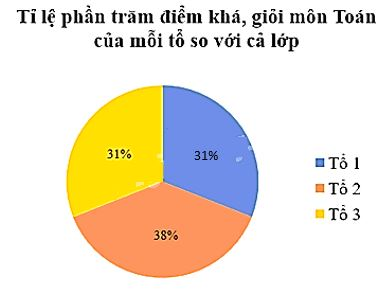–>

— *****

### Solution 6 page 115 SBT Math 7 Creative horizon episode 1 – CTST

Read the information recorded in the following pie chart and make the corresponding statistics table.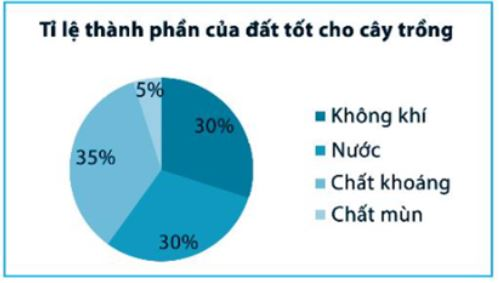Detailed instructions for solving Lesson 6

Solution method

We need to show the data contained in the chart with each data and make a statistical table

Detailed explanation

Looking at the pie chart above, we see:

– There are four components of good soil for plants represented by four fan shapes in the circle: Air, Water, Minerals, Humus.

– The percentage of each component is:

+ Percentage of Air: 30%;

+ Percentage of water accounted for: 30%;

+ Percentage of Minerals: 35%;

+ Percentage of Humus: 5%;

We have the following statistics:

 The ratio of the composition of the soil that is good for plants Ingredient Air Water Mineral Humus Ratio 30% 30% 35% 5%

–>

— *****

### Solve problems 7 pages 116 SBT Math 7 Creative horizon episode 1 – CTST

The following data table shows the number of loaves of bread sold at the canteen at Quang Trung Middle School on the past weekdays. Draw a line graph to represent this data table.

 Day Number of loaves sold at the canteen Monday 20 Tuesday 32 Wednesday 45 Thursday 36 Friday 25

Detailed instructions for solving Lesson 7

Solution method

The problem has already given the type of chart to draw, so we just need to rely on the available data in the statistics table to draw a chart with the corresponding parameters.

Detailed explanation

To draw a line graph showing the statistics of the number of loaves sold at the canteen, we perform the following steps:

Step 1: Draw two vertical and horizontal axes at right angles to each other.

– Horizontal axis: Record the timelines (Monday, Tuesday, Wednesday, Thursday, Friday).

– Vertical axis: Select the appropriate division for the data and write the numbers in the divisions.

Here we can choose a division interval of 5.

Step 2:

– At the Second datum on the horizontal axis, mark a point about 20 distances from the vertical datum at that time, corresponding to the division on the vertical axis.

The same goes for the rest of the time points.

– Draw straight lines connecting each pair of points corresponding to a pair of consecutive time points, we get a curved line representing the change of data over time.

Step 3: Complete the chart.

– Name the chart: Number of loaves sold at the canteen.

– Note the data values ​​at the beginning of the line segment.

– Record units on two axes.

We have the following chart: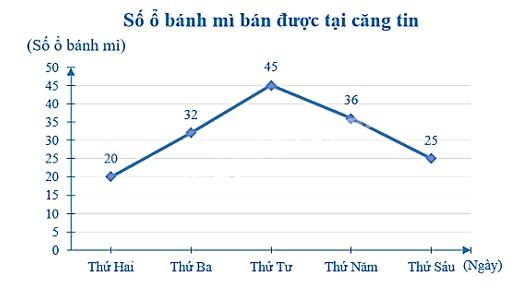–>

— *****

### Solve problems 8 pages 116 SBT Math 7 Creative horizon episode 1 – CTST

Analyze the following line graph: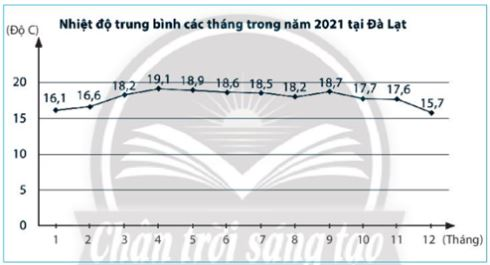Detailed instructions for solving Lesson 8

Solution method

When analyzing the chart, we need to specify the characteristics of the qualitative criteria contained in the chart. For example, in this chart, we need to indicate the month with the highest, lowest and average temperature for the whole year 2021 of Da Lat based on the chart.

Detailed explanation

Analyzing the chart above, we see:

– The chart shows the average temperature of each month in 2021 in Da Lat city.

The unit of time is the month.

– April has the highest average temperature (19.1 °C).

– December has the lowest average temperature (15.7 °C).

– Average temperature increases from January to April (from 16.1 °C to 19.1 °C) and from August to September (from 18.2 °C to 18.7 °C).

– The average temperature decreases from April to August (from 19.1 °C to 18.2 °C) and from September to December (from 18.7 °C to 15.7 °C).

–>

— *****